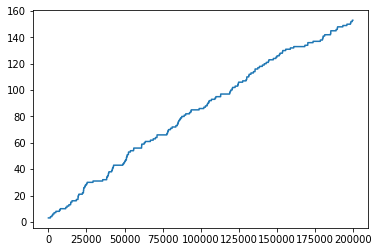# Growing polymer

In :
import numpy as np
import os

In :
readdy.__version__

Out:
'v1.0.1-0'

### Set up reaction-diffusion system¶

In :
system = readdy.ReactionDiffusionSystem(box_size=[30., 30., 30.])

In :
system.add_species("Substrate", 1.)

In :
system.topologies.configure_harmonic_bond("Head", "Core", force_constant=70, length=1.)
system.topologies.configure_harmonic_bond("Core", "Core", force_constant=70, length=1.)
system.topologies.configure_harmonic_angle("Core", "Core", "Core", force_constant=5., equilibrium_angle=np.pi)



Define reaction that is called "Bind" and attaches a "Substrate" particle to a "Polymer" topologies "Head" particle with a fixed rate once they are close enough, changing types from "Head" to "Core" and from "Substrate" to "Head".

In :
system.topologies.add_spatial_reaction(
)


### Set up simulation¶

In :
simulation = system.simulation(kernel="SingleCPU")


One initial topology with three particles (Head--Core--Head).

In :
init_top_pos = np.array([
[ 1. ,0. ,0.],
[ 0. ,0. ,0.],
[-1. ,0. ,0.]
])


Some substrate particles, randomly distributed in the simulation box.

In :
n_particles = 500
positions = np.random.uniform(size=(500,3)) * system.box_size.magnitude - system.box_size.magnitude * 0.5


Save the trajectory and topologies observable to an output file (which is removed if it already exists).

In :
simulation.output_file = 'growing_polymer.h5'
if os.path.exists(simulation.output_file):
os.remove(simulation.output_file)
simulation.observe.topologies(10)
simulation.record_trajectory(10)
simulation.progress_output_stride = 10


Run the simulation.

In :
simulation.run(200000, .001)

Configured kernel context with:
--------------------------------
- kBT = 2.43614
- periodic b.c. = (true, true, true)
- box size = (30, 30, 30)
- particle types:
* Topology particle type "Core" with D=1
*  particle type "Substrate" with D=1
* Topology particle type "Head" with D=1
- potentials of order 2:
* for types "Core" and "Core"
* Harmonic repulsion with force constant k=70
- topology potential configuration:
- bonds (2):
- Bonds for particle types Core and Core:
* Harmonic bond with force constant 70 and length 1
- Bonds for particle types Head and Core:
* Harmonic bond with force constant 70 and length 1
- angles (1):
* Harmonic angle with force constant 5 and equilibrium angle 3.14159
- topology types:
* topology type "Polymer" with 0 structural reactions
- spatial topology reactions:



In :
traj = readdy.Trajectory(simulation.output_file)


The topology_records object is a list of lists, each outer element representing a frame and each frame containing a list of topologies at that point of the simulation.

In :
chain_length = [ len(tops.particles) for tops in topology_records ]

In :
%matplotlib inline
import matplotlib.pyplot as plt
plt.plot(time, chain_length)
plt.show()